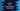# How to find the system battery information using Python## How to find the system battery information using python:

In python, we can use psutil module to read different types of system information like current battery percentage or charging status. This module can be downloaded using pip and its methods will return the different informations we need to know.

### Installing psutil:

psutil can be installed using pip:

``pip install psutil``

### sensors_battery method:

sensors_battery is the method we have to call in psutil to get the battery information. It returns None if no battery is installed. If the battery is found, it returns one tuple with the following values:

• percent: It is the battery percentage left of the battery.
• secsleft: This is the estimated seconds left of the battery before it runs out. Note that it might return POWERTIMEUNKNOWN
• power_plugged: It is True if the laptop is connected to power.

### Python program:

Let’s take a look at the below program:

``````import psutil

if __name__ == '__main__':
print(psutil.sensors_battery())``````

Here, we are printing the result of the sensors_battery method directly. It will print the below output:

``sbattery(percent=38, secsleft=14460, power_plugged=False)``

We can also print these values saperately:

``````import psutil
import datetime
from psutil._common import BatteryTime

if __name__ == '__main__':
battery_data = psutil.sensors_battery()

print('Battery power left: {}%'.format(battery_data.percent))

if battery_data.power_plugged:
print('Power is connected')
else:
print('Power is not connected')
print('Time left on battery: {}'.format(datetime.timedelta(seconds=battery_data.secsleft)))``````

It will print something like below:

``````Battery power left: 39%
Power is not connected
Time left on battery: 2:10:00``````

If it is connected to power, battery time will be POWERTIMEUNLIMITED. So, we can add one more if statement before calculating the battery time.

``````if battery_data.secsleft != BatteryTime.POWER_TIME_UNLIMITED and battery_data.secsleft != BatteryTime.POWER_TIME_UNKNOWN:
print('Time left on battery: {}'.format(datetime.timedelta(seconds=battery_data.secsleft)))``````

Also, import this:

``from psutil._common import BatteryTime``